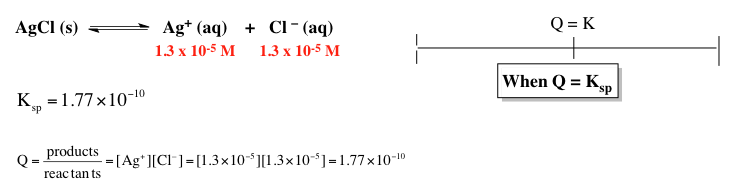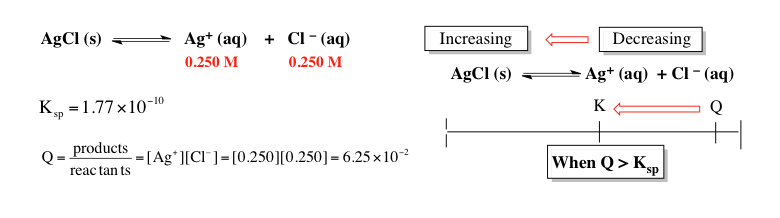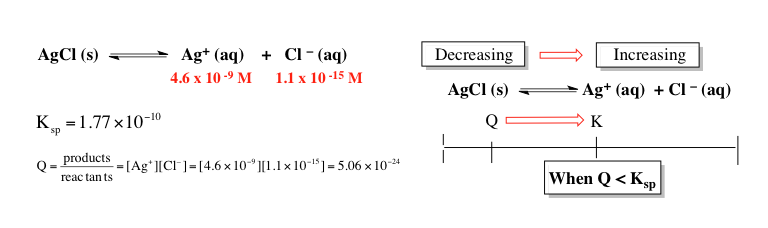Ch.6 - Chemical EquilibriumWorksheetSee all chapters

# Solubilty Product Constant

See all sections
Sections
The Equilibrium State
The Reaction Quotient
Le Chatelier's Principle
Chemical Thermodynamics: Enthalpy
Chemical Thermodynamics: Entropy
Chemical Thermodynamics: Gibbs Free Energy
Solubilty Product Constant
Protic Acids and Bases
The pH Scale
Acid Strength

The solubility product constant represents the equilibrium value for an ionic solid and its ions in a given solution.

###### Solubility Product Constant

Concept #1: The solubility product constant is a measure of how much an ionic solid will dissolve within a solvent.

Example #1: A hypothetical compound MXhas a molar solubility of 0.00562 M. What is the value of Ksp for MX3?

a) 3.16 x 10-5

b) 2.99 x 10-9

c) 9.48 x 10-5

d) 2.69 x 10-8

Example #2: Which of the following compounds will have the highest molar solubility in pure water?

a) Co(OH)2       Ksp  = 1.3 x 10-15

b) Sr3(PO4)2     Ksp  = 4.0 x 10-28

c) PbCl2           Ksp  =  1.60 x 10-5

d) AgCN          Ksp  =  5.97 x 10-17

e) PbSO4        Ksp  = 1.82 x 10-8

###### Solubility Product Constant Calculations

Example #3: La(OH)3 has a Ksp of 2.0 x 1021. How many grams of La(OH)3 (MW: 189.93 g/mol) are dissolved as hydroxide ions in 2.5 liter of a saturated solution of La(OH)3

Example #4: Find the pH of a saturated solution of Aluminum hydroxide, Al(OH)3. The Ksp of Al(OH)3 is 1.9 x 10-10.

Example #5: Calculate the molar solubility of FeCO3 in a solution of 0.00167 M Na2CO3. Ksp for FeCO3­ is 2.1 x 10-11

Practice: What is the molar solubility of Fe(OH) 3 (s) in a solution that is buffered at pH 3.50 at 25°C? The Ksp of Fe(OH)3 is 6.3 x 10–38 at 25°C.

Practice: The molar solubility of potassium dichromate, K2Cr2O7 (MW: 294.19 g/mol) is 8.3 x 10 -3 g per 100 mL solution at 20°C. Calculate its Ksp

###### The Reaction Quotient & Precipitation

Concept #2: In addition to determining if a chemical reaction is at equilibrium, the reaction quotient can also determine if a precipitate will form.

Concept #3: Comparing the reaction quotient Q to the equilibrium constant K will determine if a precipitate will form or not.

When Q is equal to Ksp the solution is saturated and there is no shifting in the equilibrium position.When Q is greater than Ksp the solution is supersaturated and the reaction shifts in reverse to form more precipitate.When Q is less than Ksp the solution is unsaturated and the reaction shifts forward to form more ions.###### The Reaction Quotient & Precipitation Calculations

Example #6: Will a precipitate form when 0.150 L of 0.100 M Pb(C2H3O2)2 and 0.100 L of 0.20 M NaCl are mixed? The Ksp value of PbCl2 is 1.2 x 10-5

Example #7: What is the minimum pH at which Fe(OH)2 will precipitate if the solution has [Fe2+] = 0.0583 M? Ksp of Fe(OH)2 is 4.87 x 10-17